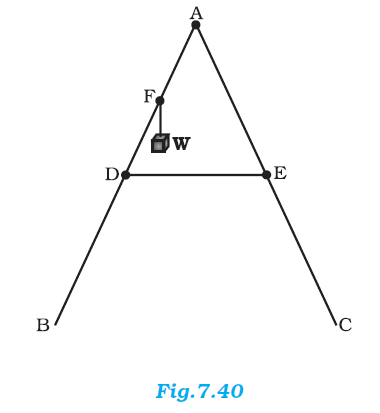## Pages

### System of particles and Rotational motion NCERT Solutions Class 11 Physics - Solved Exercise Question 7.22

Question 7.22:
As shown in Fig.7.40, the two sides of a step ladder BA and CA are 1.6 m long and hinged at A. A rope DE, 0.5 m is tied half way up. A weight 40 kg is suspended from a point F, 1.2 m from B along the ladder BA. Assuming the floor to be frictionless and neglecting the weight of the ladder, find the tension in the rope and forces exerted by the floor on the ladder. (Take g = 9.8 m/s2)
(Hint: Consider the equilibrium of each side of the ladder separately.)Solution:
The given situation can be shown as:NB = Force exerted on the ladder by the floor point B
NC = Force exerted on the ladder by the floor point C
T = Tension in the rope
BA = CA = 1.6 m
DE = 0. 5 m
BF = 1.2 m
Mass of the weight, m = 40 kg
Draw a perpendicular from A on the floor BC. This intersects DE at mid-point H.
ΔABI and ΔAIC are similar
∴BI = IC
Hence, I is the mid-point of BC.
DE || BC
BC = 2 × DE = 1 m
AF = BA – BF = 0.4 m … (i)
D is the mid-point of AB.
Hence, we can write:
AD = (1/2) X BA  =  0.8 m   ...(ii)
Using equations (i) and (ii), we get:
FE = 0.4 m
Hence, F is the mid-point of AD.
FG||DH and F is the mid-point of AD. Hence, G will also be the mid-point of AH.
ΔAFG and ΔADH are similar
∴ FG / DH  = AF / AD
FG / DH  =  0.4 / 0.8  =  1 / 2
FG = (1/2) DH
= (1/2) X 0.25  =  0.125 m

In ΔADH:
AH = (AD2 - DH2)1/2
= (0.82 - 0.252)1/2  =  0.76 m

For translational equilibrium of the ladder, the upward force should be equal to the downward force.
Nc + NB = mg = 392 … (iii)
For rotational equilibrium of the ladder, the net moment about A is:
-NB X BI + mg X FG + NC X CI + T X AG - T X AG  =  0
-NB X 0.5 + 40 X 9.8 X 0.125 + NC X 0.5  =  0
(NC - NB) X 0.5 = 49
NC - NB = 98   .....(iv)
Adding equations (iii) and (iv), we get:
NC = 245 N
NB = 147 N
For rotational equilibrium of the side AB, consider the moment about A.
-NB X BI + mg X FG + T X AG  =  0
-245 X 0.5 + 40 X 9.8 X 0.125 + T X 0.76  =  0
∴ T = 96.7 N